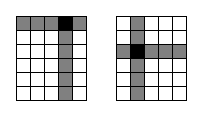ENG  RUS Timus Online JudgeOnline Judge
Problems
Authors
Online contests
Site news
Webboard
Problem set
Submit solution
Judge status
Guide
Register
Authors ranklist
Current contest
Scheduled contests
Past contests
Rules

## 1529. Game of Squares

Time limit: 5.0 second
Memory limit: 64 MB
Alice and Bob are playing with k-dimensional rectangular parallelepiped consisting of n1 × n2 × … × nk unit hypercubes. They make moves in turn. Alice chooses some unit hypercube, and cuts the parallelepiped through the center of this hypercube with all possible planes that are parallel to its sides. All unit hypercubes that were cut by at least one plane are removed, and what remains is a series of smaller rectangular parallelepipeds. It is required that at least one of those small parts has edge lengths that are pairwise relatively prime with the corresponding edge lengths of the original parallelepiped. It is also allowed to cut the parallelepiped in such way that no parts remain.
Afterwards, each player chooses any of remaining parallelepipeds, and cuts it as described above. After a cut every parallelepiped is left, and making the turn the player can choose any of them. The player who can't move loses. Assuming both players play optimally, who will win?Let's consider an example. Assume that k = 2, and we have a 6 × 5 rectangle. By cutting the hypercube at (1, 4) we get two parts: 5 × 1 and 5 × 3. As the second part's (as well as first part's, but that's not important) edge lengths are relatively prime with the edge lengths of the original rectangle (5 is relatively prime with 6 and 3 is relatively prime with 5), this is a possible move. However, cutting the hypercube at (3, 2) is not a possible move, because each of the remaining parts (2 × 1, 3 × 1, 2 × 3, 3 × 3) doesn't satisfy the condition above.

### Input

The first line of the input contains an integer k (1 ≤ k ≤ 8), the second line contains k integers n1, n2, … nk, 1 ≤ (n1+1) × (n2+1) × … × (nk+1) ≤ 10000.

### Output

Output the number of the winning player to the first line of output (1 for Alice, 2 for Bob). In case Alice wins the game, output the first move that leads her to the win to the second line of output. The move is described by k numbers, 1-based coordinates of the cut hypercube. In case there're several possible moves that lead her to the win, output the lexicographically smallest one.

### Samples

inputoutput
```2
2 2
```
```2
```
```3
3 4 5
```
```1
1 1 3
```
Problem Author: Dmitry Gozman
Problem Source: Dmitry Gozman Contest 1, Petrozavodsk training camp, January 2007